Open In App

# CSS outline-width Property

The outline is the line that is created around the specified element outside the border of the element to make the particular distinct and easier to distinguish. The outline-width Property is used to specify the width of this outline for a specific element. The outline-style property must be declared or used before the outline-width property is used on the desired element. Logically an element must have an outline for which one defines or styles the width. The outline of an element is displayed around the margin of the element and is not the same as the border property. As the outline is not a part of the element’s dimensions, therefore the element’s width and height properties do not contain the width of the outline.

Syntax:

`outline-width: medium|thin|thick|length|initial|inherit;`

Property values:

• medium: This value sets the outline width to default. The width of the outline is thinner than the outline set as thick and thicker than the outline set as thin.
• thin: This value sets the outline width to thin, the outline achieved is thinner than the outline specified with width as medium and thick.
• thick: This value sets the outline width to thick, the outline achieved is thicker than the outline specified with width as medium and think.
• length: This value is used to define the thickness of the outline.
• initial: This value sets the outline -width to its default value.
• inherit: This value inherits the specifications of the outline-width property of the parent.

Example 1: In this example, we are using outline-width: medium; property.

## html

 ```<``html``>``  ` `<``head``>``    ``<``title``>``        ``CSS | outline-width Property``    ````` `<``body``>``    ``<``p` `style="outline-width:medium;``                ``outline-style:solid;">``        ``This paragraph has medium outline.``    ``````  ` ``

Output: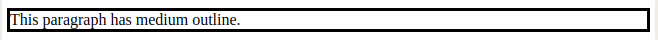Example 2: In this example, we are using outline-width: thin;

## html

 ```<``html``>``  ` `<``head``>``    ``<``title``>``        ``CSS | outline-width Property``    ````` `<``body``>``    ``<``p` `style="outline-width:thin;``              ``outline-style:solid;">``        ``This paragraph has thin outline.``    ``````  ` ``

Output: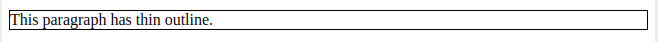Example 3: In this example, we are using outline-width: thick; property.

## html

 ```<``html``>``  ` `<``head``>``    ``<``title``>``        ``CSS | outline-width Property``    ````` `<``body``>``    ``<``p` `style="outline-width:thick;``              ``outline-style:solid;">``        ``This paragraph has thick outline.``    ``````  ` ``

Output: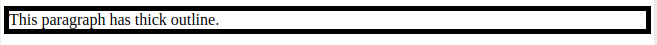Example 4: In this example, we are using the outline-width: length property.

## html

 ```<``html``>``  ` `<``head``>``    ``<``title``>``        ``CSS | outline-width Property``    ````` `<``body``>``    ``<``p` `style="outline-width:15px;``              ``outline-style:solid;">``        ``This paragraph has 15px outline.``    ``````  ` ``

Output: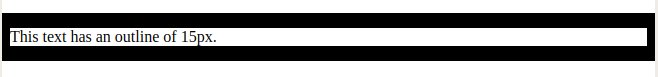Example 5: In this example, we are using outline-width: initial property.

## html

 ```<``html``>``  ` `<``head``>``    ``<``title``>``        ``CSS | outline-width Property``    ````` `<``body``>``    ``<``p` `style="outline-width:initial;``              ``outline-style:solid;">``        ``This paragraph has initial outline.``    ``````  ` ``

Output: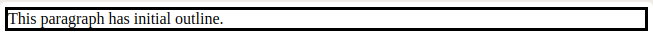Example 6: In this example, we are using outline-width: inherit property.

## html

 ```<``html``>``  ` `<``head``>``    ``<``title``>``        ``CSS | outline-width Property``    ````` `<``body``>``    ``<``p` `style="outline-width:medium;``              ``outline-style:solid;">``        ``This paragraph has medium outline.``    ``` `    ``<``p` `style="outline-width:thin;``              ``outline-style:solid;">``        ``This paragraph has thin outline.``    ````    ``<``br``>` `    ``<``p` `style="outline-width:thick;``              ``outline-style:solid;">``        ``This paragraph has thick outline.``    ````    ``<``br``>` `    ``<``p` `style="outline-width:15px;``                ``outline-style:solid;">``        ``This text has an outline of 15px.``    ````    ``<``br``><``br``>``    ``<``p` `style="outline-width:initial;``              ``outline-style:solid;">``        ``This text has an outline of default``        ``type set by initial``    ``` ```  ` ``

Output: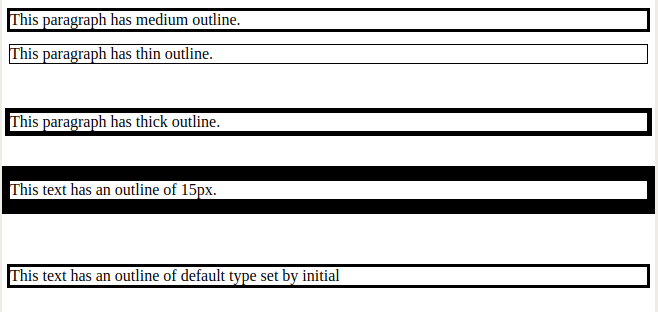Supported Browsers: The browser supported by CSS | outline-width Property are listed below:

• Google Chrome 1.0 and above
• Edge 12.0 and above
• Firebox 1.5 and above
• Opera 7.0 and above
• Safari 1.2 and above